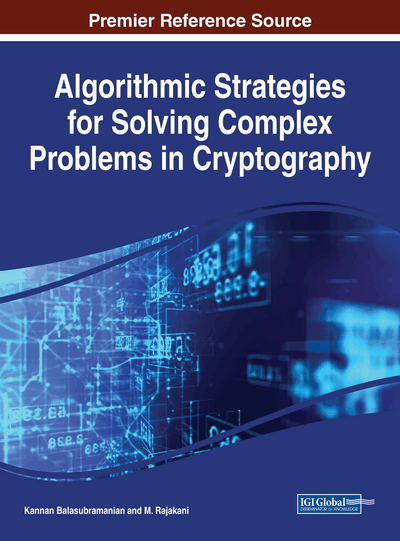# Integer Factoring Algorithms

Kannan Balasubramanian (Mepco Schlenk Engineering College, India) and Ahmed Mahmoud Abbas (The American University in Cairo, Egypt)
Copyright: © 2018 |Pages: 13
DOI: 10.4018/978-1-5225-2915-6.ch017
OnDemand PDF Download:
Available
\$37.50
No Current Special Offers

## Abstract

Most cryptographic systems are based on an underlying difficult problem. The RSA cryptosystem and many other cryptosystems rely on the fact that factoring a large composite number into two prime numbers is a hard problem. The are many algorithms for factoring integers. This chapter presents some of the basic algorithms for integer factorization like the Trial Division, Fermat's Algorithm. Pollard's Rho Method, Pollard's p-1 method and the Elliptic Curve Method. The Number Field Sieve algorithm along with Special Number field Sieve and the General Number Field Sieve are also used in factoring large numbers. Other factoring algorithms discussed in this chapter are the Continued Fractions Algorithms and the Quadratic Sieve Algorithm.
Chapter Preview
Top

## The Factorization Problem

Factoring a positive integer n means finding positive integers u and v such that the product of u and v equals n, and such that both u and v are greater than 1. Such u and v are called factors (or divisors) of n, and n = u . v is called a factorization of n. Positive integers that can be factored are called composites. Positive integers greater than 1 that cannot be factored are called primes. A factorization of a composite number is not necessarily unique. But the prime factorization of a number—writing it as a product of prime numbers—is unique, up to the order of the factors.

We are interested in finding just a factorization. The prime factorization can be obtained by further factoring the factors that happen to be composite. Factoring a composite integer is believed to be a hard problem. This is, of course, not the case for all composites—composites with small factors are easy to factor—but, in general, the problem seems to be difficult. As yet there is no firm mathematical ground on which this assumption can be based. The only evidence that factoring is hard consists of our failure so far to find a fast and practical factoring algorithm.

This relation between factoring and cryptography is one of the main reasons why people are interested in evaluating the practical difficulty of the integer factorization problem. Currently the limits of our factoring capabilities lie around 130 decimal digits (Lenstra, 2000). Factoring hard integers in that range requires enormous amounts of computing power. A cheap and convenient way to get the computing power needed is to distribute the computation over the Internet. This approach was first used in 1988 to factor a 100-digit integer (Lenstra, 1990), since then to factor many integers in the 100 to 120 digit range, and in 1994 to factor the famous 129-digit RSA-challenge number. Most recently, in 1996 a 130-digit number was factored, partially using a World Wide Web interface (Cowie et.al., 1996).

In this chapter, we illustrate the basic steps involved in the factoring methods used to obtain the factorizations just mentioned and we explain how these methods can be run in parallel on a loosely coupled computer network, such as the Internet. We distinguish two main types of factoring methods: those that work quickly if one is lucky, and those that are almost guaranteed to work no matter how unlucky one is. The latter are referred to as general-purpose algorithms and have an expected run time that depends solely on the size of the number n being factored. The former are called special-purpose algorithms; they have an expected run time that also depends on the properties of the— unknown—factors of n. When evaluating the security of factoring-based cryptosystems, people employ general-purpose factoring algorithms.

Top

## Special Purpose Factoring Algorithms

We briefly discuss five of the most important special purpose factoring methods: trial division, Pollard’s rho method, Pollard’s p- 1 method, the elliptic curve method and Fermat’s method. We assume that n denotes the number to be factored. We also assume that n is composite and not a prime power.

## Complete Chapter List

Search this Book:
Reset